# 0708-1300/Homework Assignment 12

(diff) ← Older revision | Latest revision (diff) | Newer revision → (diff)
Jump to: navigation, search
Announcements go here

## Reading

Read, reread and rereread your notes to this point, and make sure that you really, really really, really really really understand everything in them. Do the same every week! Also, read section 8-11, 13 and 18-20 of chapter IV of Bredon's book (three times, as always).

## Doing

Solve all the problems in pages 206-207 of Bredon's book, but submit only your solutions of problems 1, 5, 9, and all the problems in pages 230 but submit only problem 1. Also, solve and submit the following:

Problem 12. Given a CW-space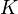$K$ with$n$-cells indexed by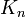$K_n$ and skeleta denoted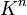$K^n$, show that the map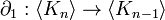$\partial_1:\langle K_n\rangle\to\langle K_{n-1}\rangle$ given by the composition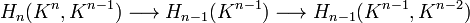$H_n(K^n, K^{n-1})\longrightarrow H_{n-1}(K^{n-1})\longrightarrow H_{n-1}(K^{n-1}, K^{n-2})$ is equal to the one defined using degrees: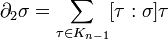$\partial_2\sigma=\sum_{\tau\in K_{n-1}}[\tau:\sigma]\tau$, where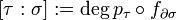$[\tau:\sigma]:=\deg p_\tau\circ f_{\partial\sigma}$ and the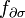$f_{\partial\sigma}$'s are the gluing maps defining$K$.

Hint. Dror's notes on the subject are:

## Due Date

This assignment is due in class on Thursday April 10, 2008.